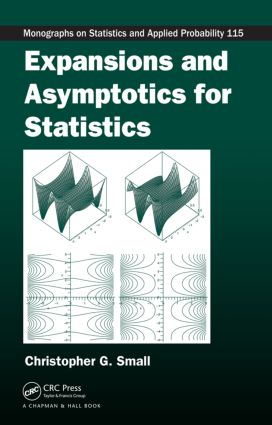# Expansions and Asymptotics for Statistics

## 1st Edition

Chapman and Hall/CRC

357 pages | 28 B/W Illus.

Hardback: 9781584885900
pub: 2010-05-07
SAVE ~\$25.00
\$125.00
\$100.00
x
eBook (VitalSource) : 9780429147593
pub: 2010-05-07
from \$28.98

FREE Standard Shipping!

### Description

Asymptotic methods provide important tools for approximating and analysing functions that arise in probability and statistics. Moreover, the conclusions of asymptotic analysis often supplement the conclusions obtained by numerical methods. Providing a broad toolkit of analytical methods, Expansions and Asymptotics for Statistics shows how asymptotics, when coupled with numerical methods, becomes a powerful way to acquire a deeper understanding of the techniques used in probability and statistics.

The book first discusses the role of expansions and asymptotics in statistics, the basic properties of power series and asymptotic series, and the study of rational approximations to functions. With a focus on asymptotic normality and asymptotic efficiency of standard estimators, it covers various applications, such as the use of the delta method for bias reduction, variance stabilisation, and the construction of normalising transformations, as well as the standard theory derived from the work of R.A. Fisher, H. Cramér, L. Le Cam, and others. The book then examines the close connection between saddle-point approximation and the Laplace method. The final chapter explores series convergence and the acceleration of that convergence.

### Reviews

This book will be an excellent resource for researchers and graduate students who need a deeper understanding of functions arising in probability and statistics than that provided by numerical techniques.

—Eduardo Gutiérrez-Peña, International Statistical Review, 2012

This outstanding book is rich in contents and excellent in readability. … I enjoyed reading this book and found this book valuable in my research as well as in my understanding of expansions and asymptotics as they arise often in statistics. The author has to be commended for his contribution to our profession in getting this book out.

—Subir Ghosh, Technometrics, May 2012

I have found this book very useful not only for the specialists in asymptotics but especially for all those who wish to learn more from this field and to see the inter-relations between different approaches.

—Jaromir Antoch, Zentralblatt MATH

This is an excellent book for researchers interested in asymptotics, especially those working on (mathematical) statistics or applied probability. … The book contains a compilation of different techniques to deal with series expansions and approximations with statistical applications. Examples are focused on the approximation of probability densities, distributions and likelihoods.

—Javier Carcamo, Mathematical Reviews

Introduction

Expansions and approximations

The role of asymptotics

Mathematical preliminaries

Two complementary approaches

General Series Methods

A quick overview

Power series

Enveloping series

Asymptotic series

Superasymptotic and hyperasymptotic series

Asymptotic series for large samples

Generalised asymptotic expansions

Notes

Padé approximations for the exponential function

Two applications

Continued fraction expansions

A continued fraction for the normal distribution

Approximating transforms and other integrals

Multivariate extensions

Notes

The Delta Method and Its Extensions

Introduction to the delta method

Preliminary results

The delta method for moments

Using the delta method in Maple

Asymptotic bias

Variance stabilising transformations

Normalising transformations

Parameter transformations

Functions of several variables

Ratios of averages

The delta method for distributions

The von Mises calculus

Obstacles and opportunities: robustness

Optimality and Likelihood Asymptotics

Historical overview

The organisation of this chapter

The likelihood function and its properties

Consistency of maximum likelihood

Asymptotic normality of maximum likelihood

Asymptotic comparison of estimators

Local asymptotics

Local asymptotic normality

Local asymptotic minimaxity

Various extensions

The Laplace Approximation and Series

A simple example

The basic approximation

The Stirling series for factorials

Laplace expansions in Maple

Asymptotic bias of the median

Recurrence properties of random walks

Proofs of the main propositions

Integrals with the maximum on the boundary

Integrals of higher dimension

Integrals with product integrands

Applications to statistical inference

Estimating location parameters

Asymptotic analysis of Bayes estimators

Notes

The principle of stationary phase

Towards the Barndorff–Nielsen formula

Ratios of sums of random variables

Distributions of M-estimators

The Edgeworth expansion

Mean, median and mode

The method of Darboux

Applications to common distributions

Summation of Series

Convergence of random series

Applications in probability and statistics

Euler–Maclaurin sum formula

Applications of the Euler–Maclaurin formula

Accelerating series convergence

Applications of acceleration methods

Comparing acceleration techniques

Divergent series

Glossary of Symbols

Useful Limits, Series and Products

References

Index